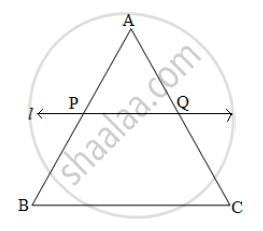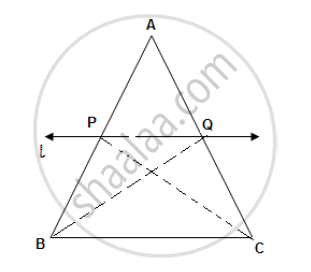# In a triangle ABC, line l || Side BC and line l intersects side AB and AC in points P and Q, respectively. Prove that: AP/BP=AQ/QC - Geometry

In a triangle ABC, line l || Side BC and line l intersects side AB and AC in points P and Q, respectively. Prove that: "AP"/"BP"="AQ"/"QC"#### Solution

Construction: Join seg PC and seg BQ.Given: In ΔABC , line l || BC

Line l intersects side AB and side AC in points P and Q, respectively.

A-P-B and A-Q-C

"To Prove: ""AP"/"BP"="AQ"/"QC"

Construction: Join seg BQ and seg CP

In ΔAPQ and ΔBPQ ,

(A(triangleAPQ))/(A(triangleBPQ))=(AP)/(BP)  "          (i) ...........""(Triangles having equal height)"

In ΔAPQ and ΔCPQ

(A(triangleAPQ))/(A(triangleCPQ))=(AQ)/(CQ)"             (ii) ......(Triangles having equal height)"

A(triangleBPQ)=A(triangleCPQ)"           (iii) .....(Triangles with common base PQ and same height)"

(A(triangleAPQ))/(A(triangleBPQ))=(A(triangleAPQ))/(A(triangleCPQ))"             ..............From (i), (ii) and (iii)"

"AP"/"BP"="AQ"/"QC"

Concept: Similarity of Triangles
Is there an error in this question or solution?